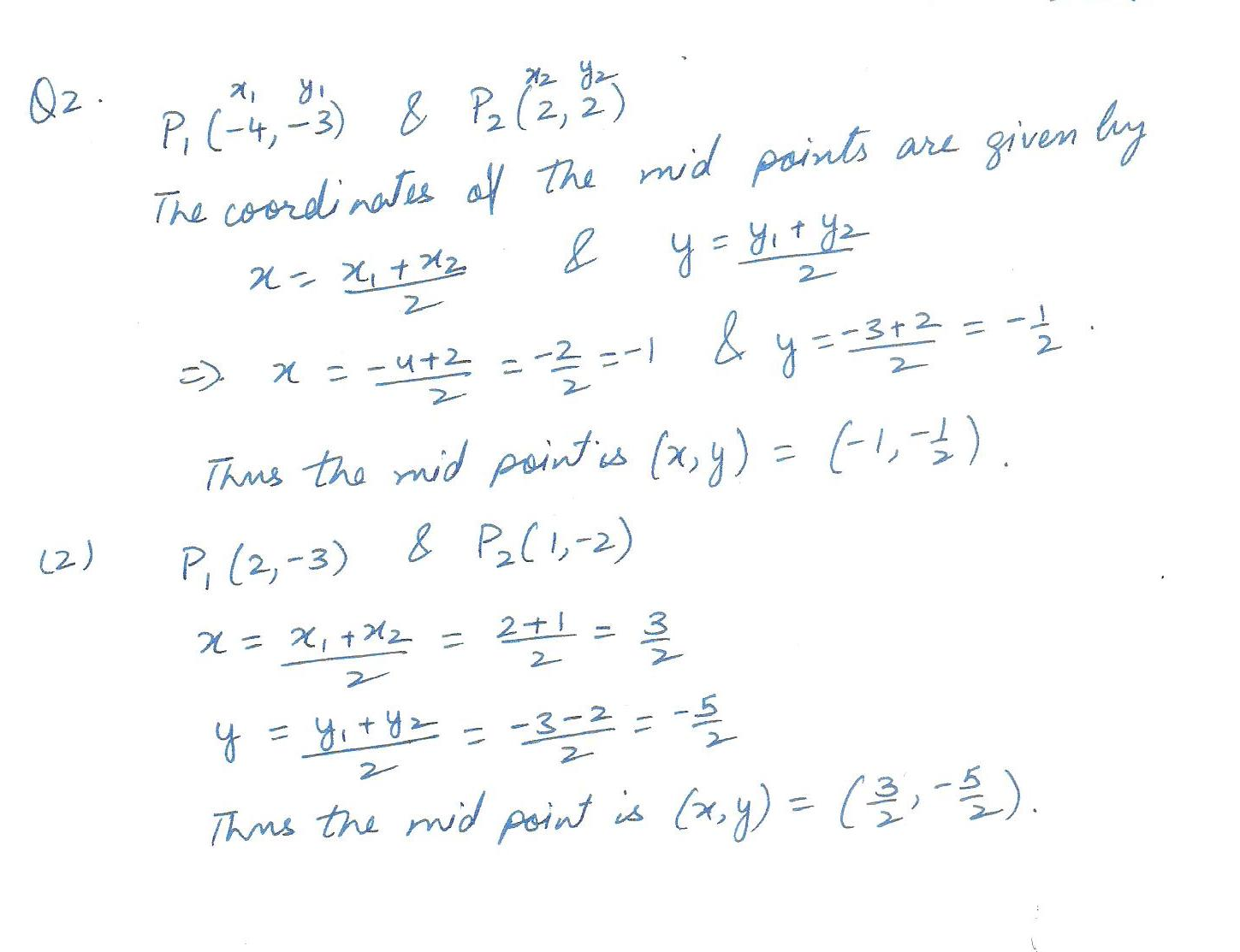6248

Mathematics Coordinate Geometry Level: Misc Level

Looking for the midpoint between the line segment of the points these 2 problems:

1) P1=(-4,-3) andP2=(2,2) (2) P1=(2,-3) and P2=(1,-2)